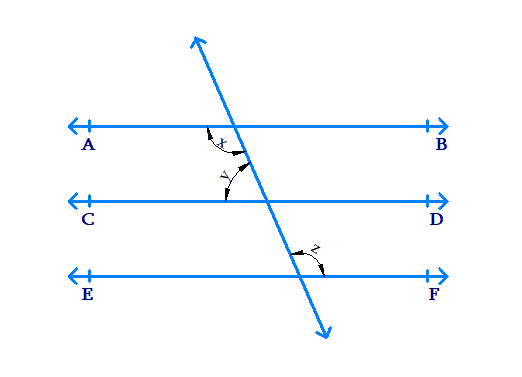# Ex.6.2 Q2 Lines and Angles Solution - NCERT Maths Class 9

Go back to  'Ex.6.2'

## Question

In the given figure, if $$AB\, ‖ \,CD\,,\; CD\, ‖ \,EF$$ and $$y:z = 3:7$$, find $$x$$.Video Solution
Lines And Angles
Ex 6.2 | Question 2

## Text Solution

What is known?

$$AB ‖ CD$$

$$CD ‖ EF$$

$$y:z = 3:7$$ implies $$y = 3a$$ and $$z = 7a$$

What is unknown?

Value of $$x.$$

Reasoning:

• Lines which are parallel to the same line are parallel to each other.
• When two parallel lines are cut by a transversal, co-interior angles formed are supplementary.

Steps:

We know that, lines which are parallel to the same line are parallel to each other.

If $$AB ‖ CD$$, $$CD ‖ EF$$, we can say that $$AB ‖ EF$$.

Therefore, the angles $$x$$ and $$z$$ are alternate interior angles and hence are equal.

$x = z \qquad\ldots \ldots \ldots (1)$

$$AB$$ and $$CD$$ are parallel lines cut by transversal. So the co-interior angles formed are supplementary.

$x + y =180^ {\circ}$

$$\text{Since}\ x = z$$, we get $$y + z =180^ {\circ}$$

Given $$y = 3a, \;z = 7a$$

\begin{align} 3 a + 7 a &= 180 ^ { \circ } \\ 10 a &= 180 ^ { \circ } \\ a &= \frac { 180^ { \circ } } { 10 } \\ a &= 18 ^ { \circ } \end{align}

\begin{align} \therefore \qquad y & = 3 a \\ y &= 3 \times 18 ^ { \circ } \\ y &= 54 ^ { \circ } \end{align}

\begin{align} \therefore \qquad x + y &= 180 ^ { \circ } \\ x + 54 ^ { \circ } &= 180 ^ { \circ } \\ x &= (180 ^ { \circ } - 54 ^ { \circ }) \\ x &= 126 ^ { \circ } \end{align}

Video Solution
Lines And Angles
Ex 6.2 | Question 2

Learn from the best math teachers and top your exams

• Live one on one classroom and doubt clearing
• Practice worksheets in and after class for conceptual clarity
• Personalized curriculum to keep up with school